﻿ C++调用python基本数据类型实例--锲而不舍的trying - 豆末

## C++调用python基本数据类型实例--锲而不舍的tryingps：本废柴少女不仅废柴，还懒。So直接从我的CSDN账号里原封不动地copy过来了...

okay，to be serious now

----------------------------------------------------------------------------------------------------------这是一条严肃的分割线

C++调用Python实例
C++调用Python浅析

Okay下面开始

## 1.新建项目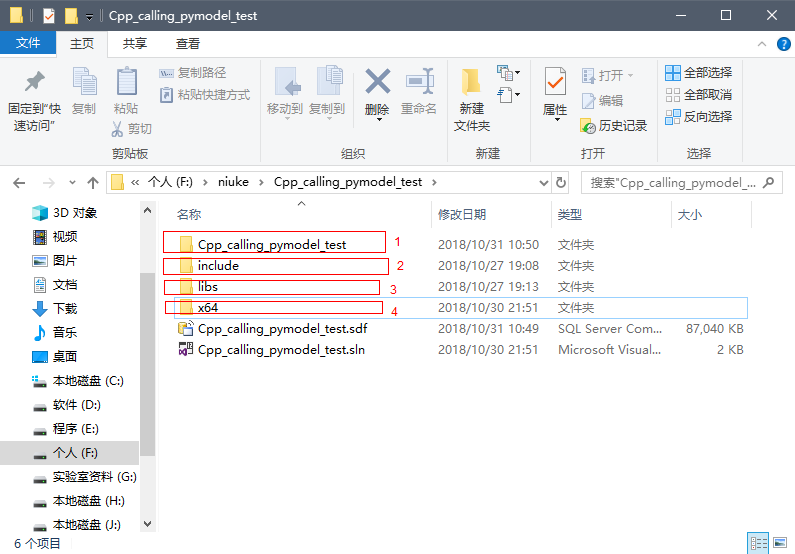## 2.配置属性管理器

C/C++ -->常规 -->附加包含目录 -->添加 F:niukeCpp_calling_pymodel_testinclude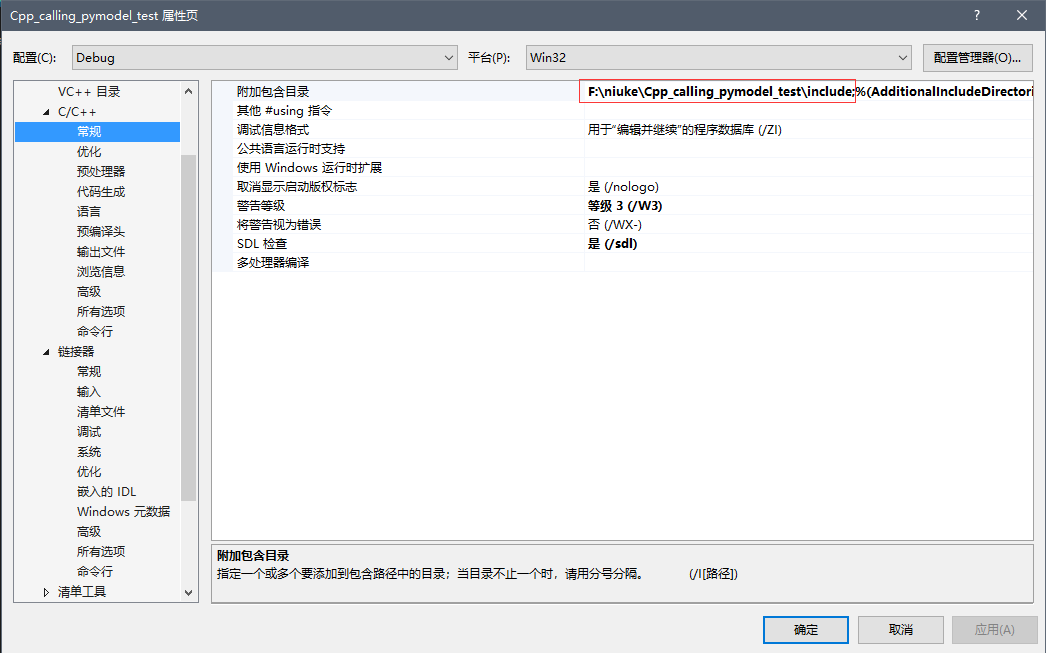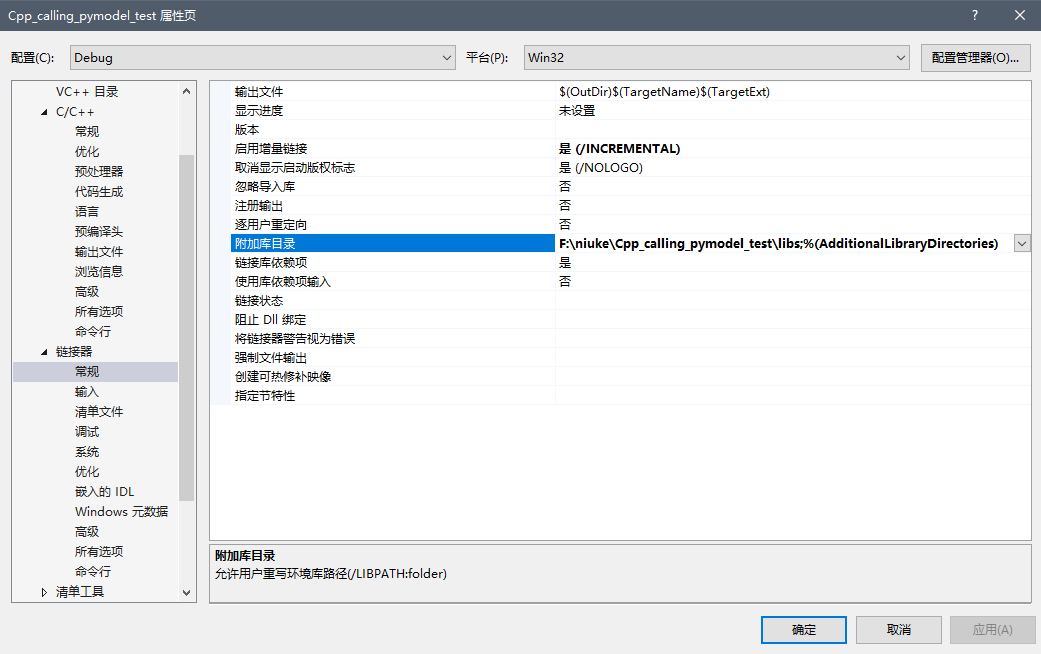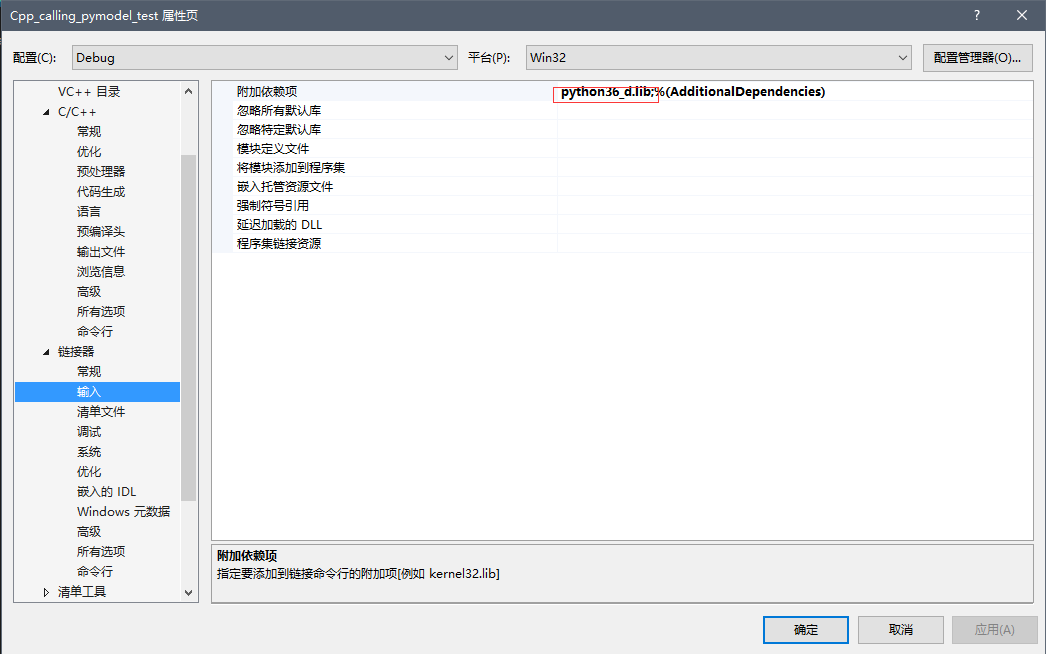## 3.其他

Anaconda3文件夹下的python36.dll文件拷贝到Debug目录下（与Cpp_calling_pymodel_test.exe 同目录）。在该Debug目录下新建一个.txt文件，将其命名为Test_origin.py（具体内容详见后面）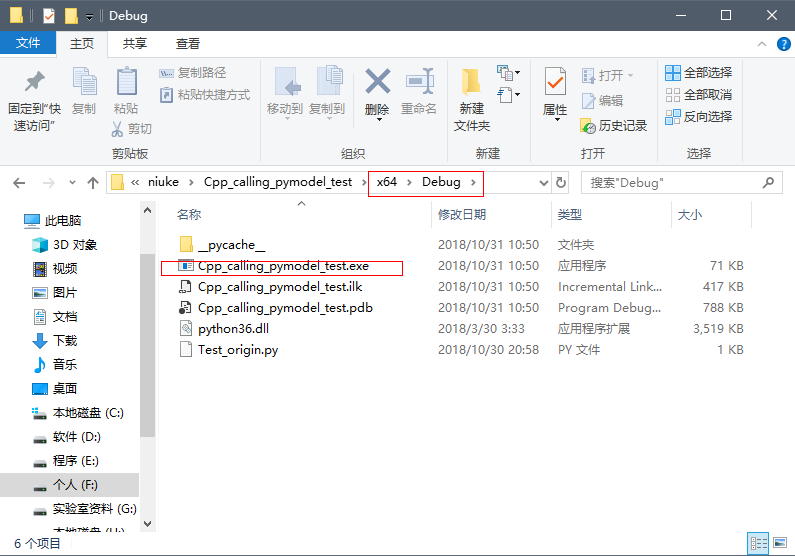## 4.挂代码

``````#include <iostream>
using namespace std;
extern "C"
{
#include "Python.h"
}
//调用输出"Hello Python"函数
void Hello()
{
Py_Initialize();//调用Py_Initialize()进行初始化
PyObject * pModule = NULL;
PyObject * pFunc = NULL;
pModule = PyImport_ImportModule("Test001");//调用的Python文件名
pFunc = PyObject_GetAttrString(pModule, "Hello");//调用的函数名
PyEval_CallObject(pFunc, NULL);//调用函数,NULL表示参数为空
Py_Finalize();//调用Py_Finalize,和Py_Initialize相对应的.
}
//调用Add函数,传两个int型参数
void Add()
{
Py_Initialize();
PyObject * pModule = NULL;
PyObject * pFunc = NULL;
pModule = PyImport_ImportModule("Test001");//Test001:Python文件名
pFunc = PyObject_GetAttrString(pModule, "Add");//Add:Python文件中的函数名
//创建参数:
PyObject *pArgs = PyTuple_New(2);//函数调用的参数传递均是以元组的形式打包的,2表示参数个数
PyTuple_SetItem(pArgs, 0, Py_BuildValue("i", 6));//0--序号,i表示创建int型变量
PyTuple_SetItem(pArgs, 1, Py_BuildValue("i", 8));//1--序号
//返回值
PyObject *pReturn = NULL;
pReturn = PyEval_CallObject(pFunc, pArgs);//调用函数
//将返回值转换为int类型
int result;
PyArg_Parse(pReturn, "i", &result);//i表示转换成int型变量
cout << "6 + 8 = " << result << endl;
Py_Finalize();
}
int main(int argc, char** argv)
{
cout << "调用Test001.py中的Hello函数..." << endl;
Hello();
cout << "\n调用Test001.py中的Add函数..." << endl;
Add();
getchar();
return 0;
}``````

Test_origin.py

``````def Hello():
print("Hello Python")

def Add(a, b):
return a+b``````

----------------------------------------------------------------------------------------------------------这是一条严肃的分割线

## 5.#悲催的后续

C++调用python
C++调用python配置及编译出现的问题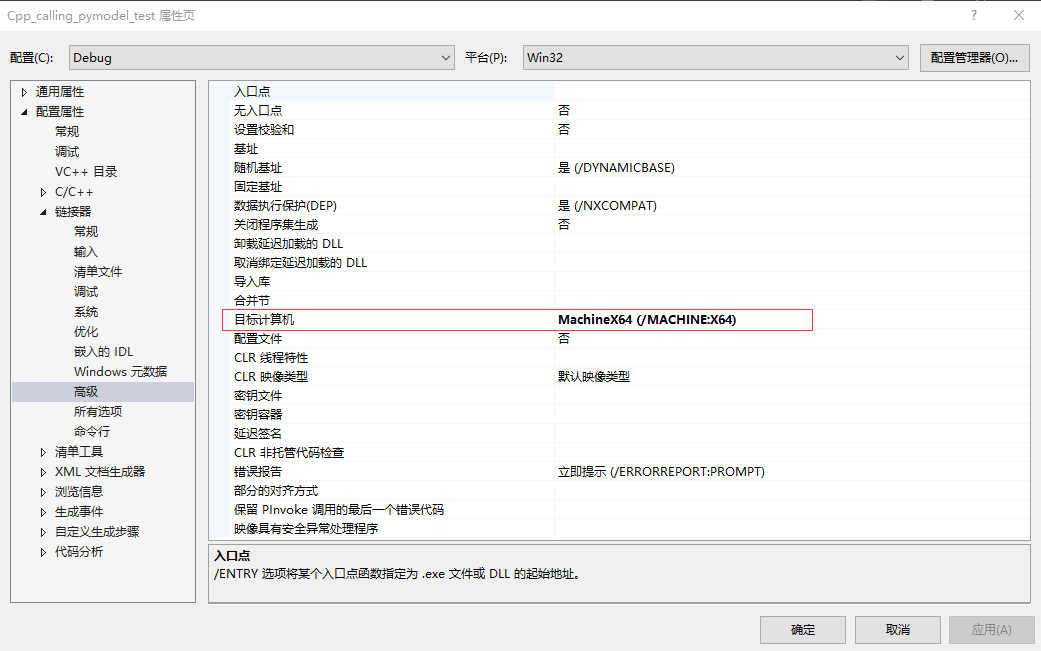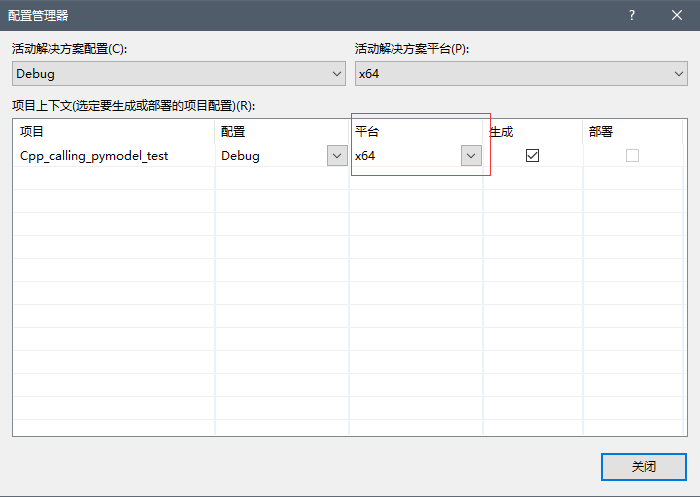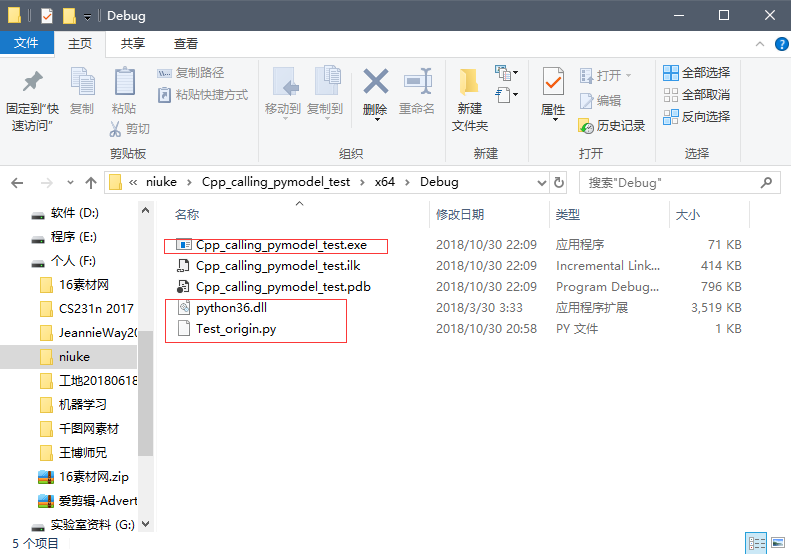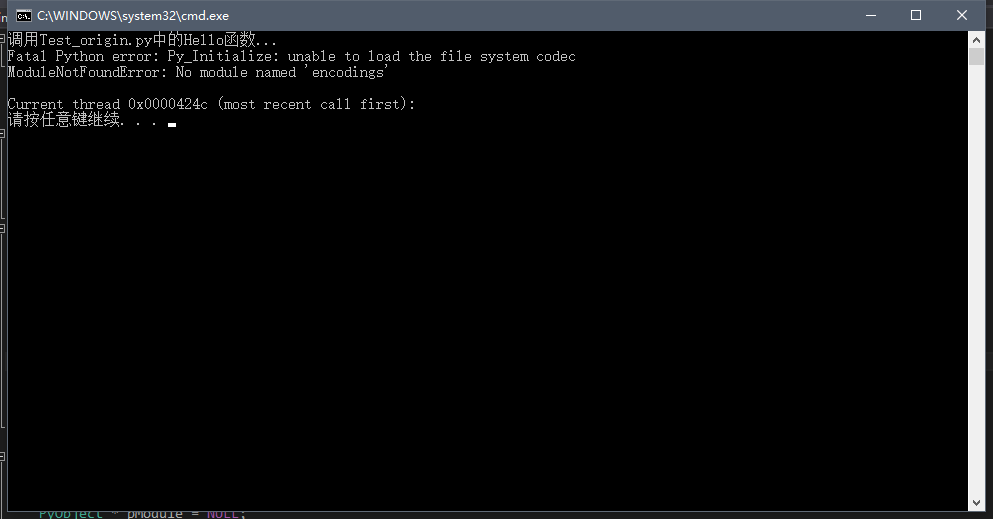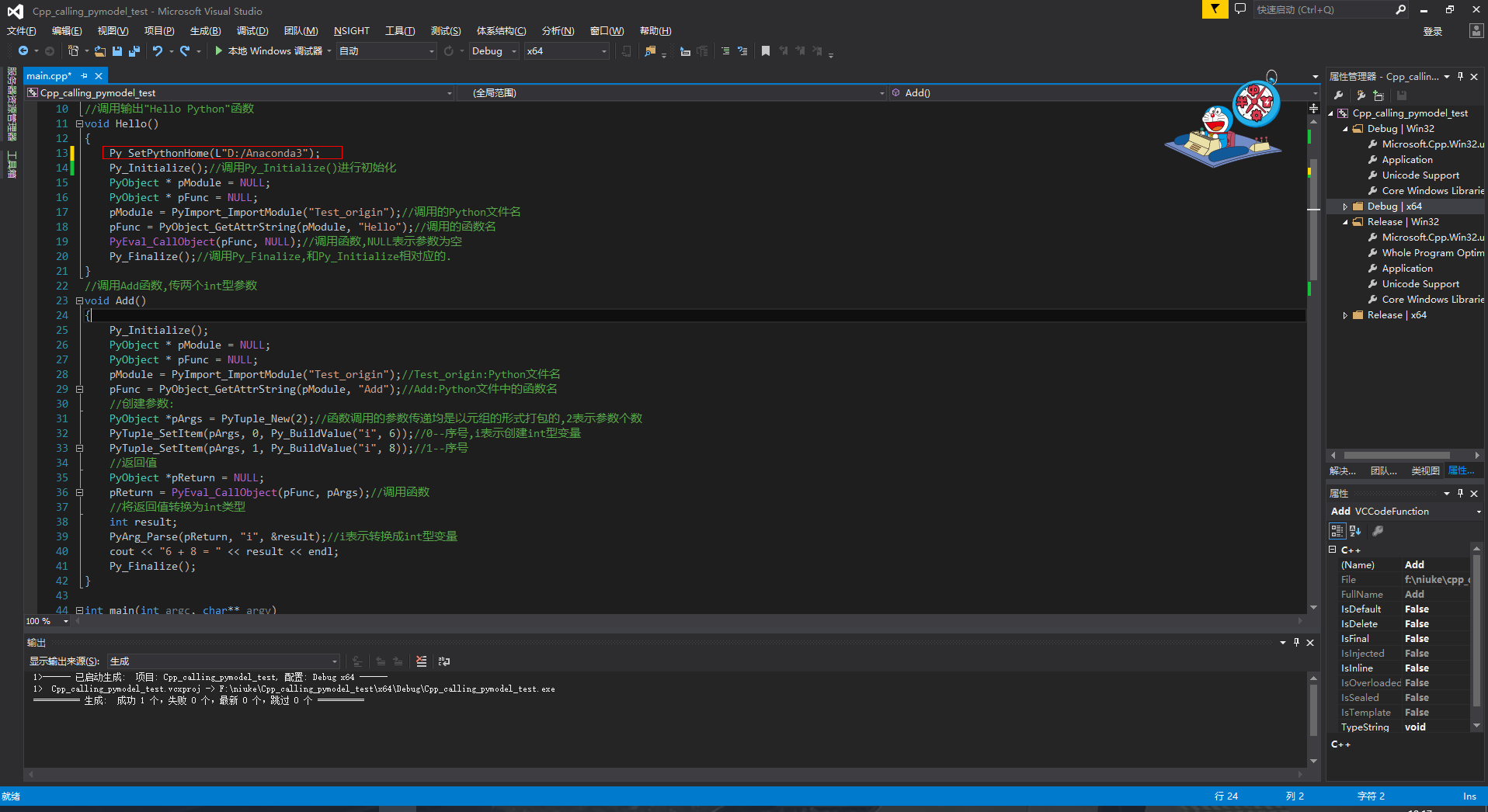``Py_SetPythonHome(L"D:/Anaconda3");``

Okay终于完美解决~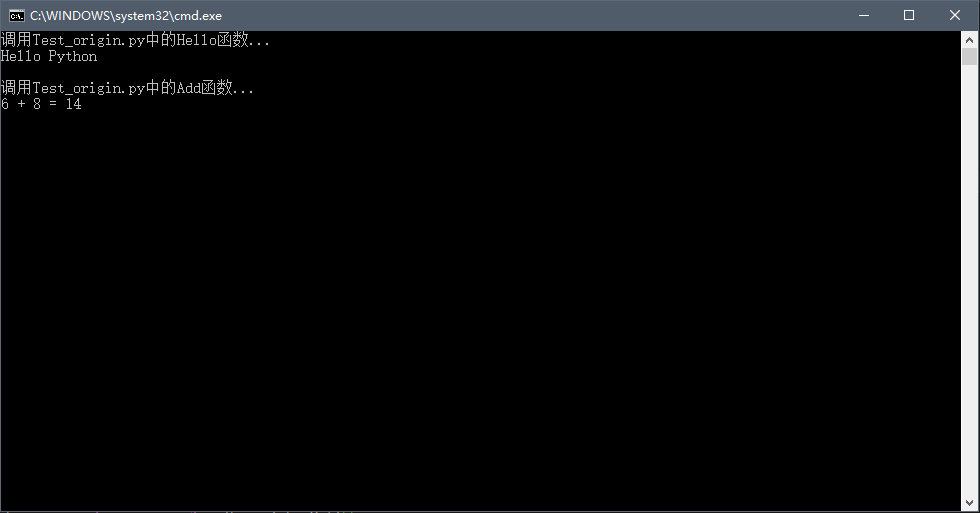© 著作权归作者所有

*选项为必填